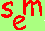David A. Kenny
October 29, 2011

Single Latent Variable Model

Representation in a Path Model
The latent variable or factor is usually represented by a oval.
Measured variables or indicators are represented by a rectangle.

Standard Assumptions
The latent variable is uncorrelated with the errors of measurement in each of the indicators.
The errors of different indicators are uncorrelated with each other.

Parameters
loadings: the effect of latent variable on the measure; if a measure loads on only one latent variable, the standardized loading is the measure's correlation with the latent variable and can be interpreted as the square root of the measure's reliability.
error variance:the variance in the indicator not explained by the latent variable; error variance does not imply that the variance is random or not meaningful, just that it is unexplained by the latent variable

Identification Rules
at least three indicators of the latent variable with no correlated errors or two loadings are set equal to each other (e.g., both equal to one)
restriction to achieve identification
unstandardized models:  one of the loadings is set to one (called the marker variable), the others are free, and the latent variable variance is free (Go to a discussion of standardization in structural equation models.)
standardized models:  the latent variable's variance is set equal to one and all the loadings are free to vary

Problems in Estimation
Heywood Cases
definition: the standardized loading is larger than one and the error variance is negative
possible solutions to the problem:
1) Treat as specification error and modify the model.
2) Create a non-linear constraint on the loading to prevent it from being too large or prevent the error variance from being negative.
3) Fix the standardized loading to one (usually one is subtracted from the degrees of freedom outputted by computer programs).
Empirical Underidentification (to learn about empirical underidentification)
weak correlations: the correlation between all pairs of indicators is not significantly different from zero
for three indicators, the product of the three correlations is negative
the marker variable does not correlate with the other indicators

Conversion from the Standardized to the Unstandardized Solution (Go to a discussion of standardization in structural equation models.)
latent variable variance: multiply the squared loading of the marker variable times the variance of the marker